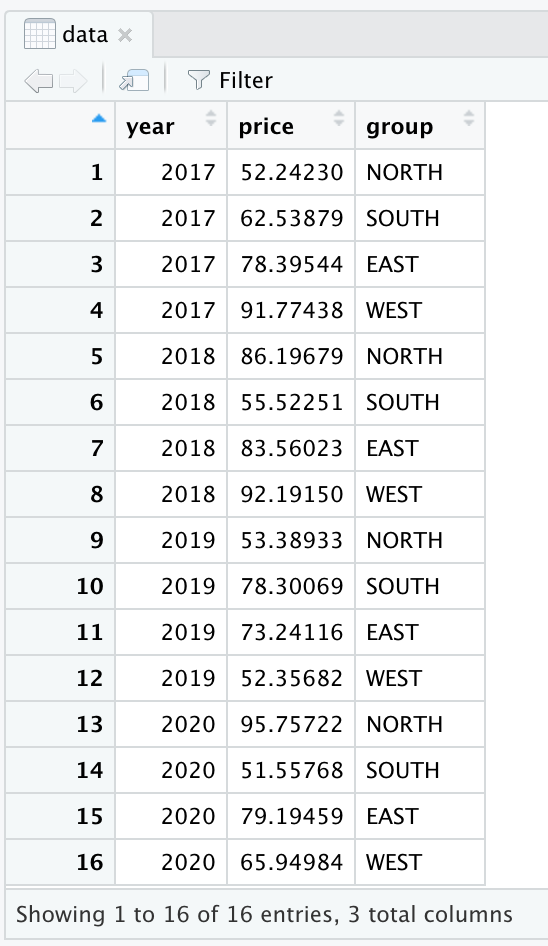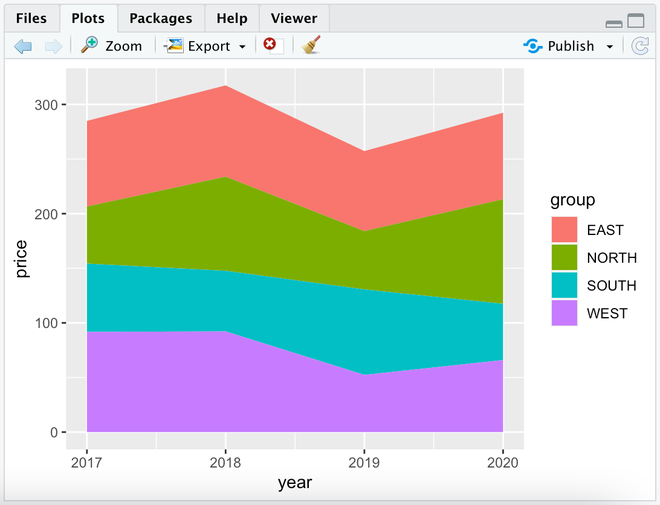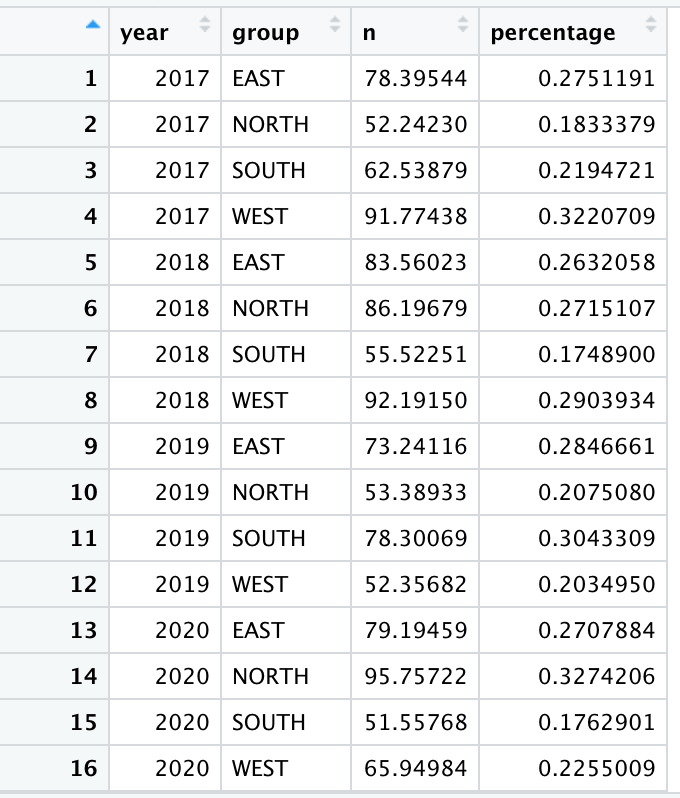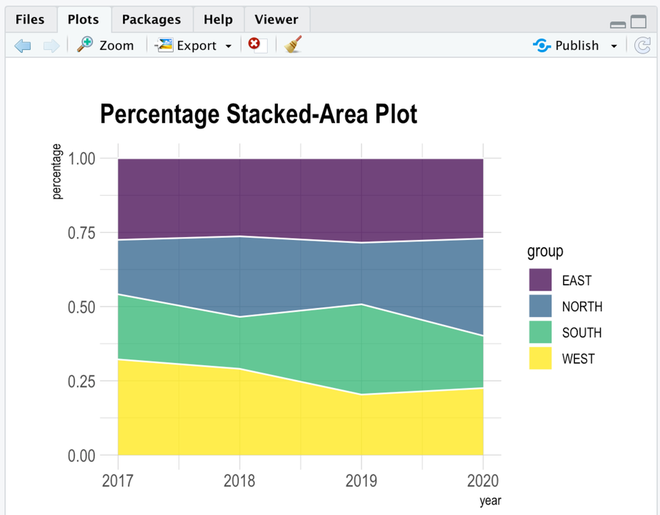Related Articles

# Stacked area chart with R

• Last Updated : 16 Mar, 2021

Area Charts are defined as the filled regions between two series that share common areas. A stacked area chart is easily understandable if you know the area chart. This plot display evolution of cost/value of several groups on the same plot. The values of each group are displayed on top of every other. Using this plot, we can analyze the total numerical value of variables present in each group section-wise and the importance of each group.

R uses the function geom_area() to create Stacked area charts.

Syntax: ggplot(Data, aes(x=x_variable, y=y_variable, fill=group_variable)) + geom_area()

Parameters:

• Data: This parameter contains whole dataset which are used in stacked-area chart.
• x: This parameter contains numerical value of variable for x axis in stacked-area chart.
• y: This parameter contains numerical value of variables for y axis in stacked-area chart.
• fill: This parameter contains group column of Data which is mainly used for analyse in stacked-area chart.

Creating a basic Stacked area chart:

Step 1: Import Packages

## R

 `library``(ggplot2)``library``(dplyr)``library``(tidyverse)`

Step 2: Create Dataset

In the Group column, Four Direction is replicated 4 times. Year column, the sequence is generated from 2017 to 2020, each 4 times. Price column, generated by runif(n, min, max) function.

## R

 `group <- ``rep``(``c``(``"NORTH"``,``"SOUTH"``,``"EAST"``,``"WEST"``),times=4)  ``year <- ``as.numeric``(``rep``(``seq``(2017,2020),each=4)) ``price <- ``runif``(16, 50, 100)`` ` `data <- ``data.frame``(year, price, group)`

Output:Step 3: Plot the Data

## R

 `ggplot``(data, ``aes``(x=year, y=price, fill=group)) + ``geom_area``()`

Output:## Percentage stacked-area chart

In the basic stacked-area chart, if we interested in the relative interest of each group, then we can draw a percentage stacked-area chart. This plot will normalize our data then plot. This can be done using dplyr library.

Step 1: Calculate the percentage

## R

 `data <- data  %>%``+     ``group_by``(year, group) %>%``+     ``summarise``(n = ``sum``(price)) %>%``+     ``mutate``(percentage = n / ``sum``(n))`

Output:Step 2: Plot data

We can add color in a plot by Viridis library, Title by ggtitle, and theme_ipsum of the hrbrthemes package

## R

 `library``(viridis)``library``(hrbrthemes)`` ` `ggplot``(data, ``aes``(x=year, y=percentage, fill=group)) ``+     ``geom_area``(alpha=0.8 , size=.5, colour=``"white"``) ``+    ``scale_fill_viridis``(discrete = T) ``+    ``theme_ipsum``() ``+     ``ggtitle``(``"Percentage Stacked-Area Plot"``)`

Output:My Personal Notes arrow_drop_up